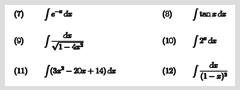# Home | Mathematics | Integral

## Integral

In mathematics, an integral assigns numbers to functions in a way that can describe displacement, area, volume, and other concepts that arise by combining infinitesimal data. Integration is one of the two main operations of calculus, with its inverse operation, differentiation, being the other. Given a function f of a real variable x and an interval [a, b] of the real line, the definite integralIntegration – Simple exampleTrigonometric polynomials